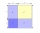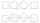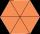# Area of shape + ratio - math problems

#### Number of problems found: 70

• Railway embankmentThe section of the railway embankment is an isosceles trapezoid, the sizes of the bases of which are in the ratio 5: 3. The arms have a length of 5 m and the height of the embankment is 4.8 m. Calculates the size of the embankment section area.
• Sphere in coneA sphere is inscribed in the cone (the intersection of their boundaries consists of a circle and one point). The ratio of the surface of the ball and the contents of the base is 4: 3. A plane passing through the axis of a cone cuts the cone in an isoscele
• The circumferenceThe circumference and width of the rectangle are in a ratio of 5: 1. its area is 216cm2. What is its length?
• Garden exchangeThe garden has the shape of a rectangular trapezoid, the bases of which have dimensions of 60 m and 30 m and a vertical arm of 40 m. The owner exchanged this garden for a parallelogram, the area of which is 7/9 of the area of a trapezoidal garden. What is
• Squares ratioThe first square has a side length of a = 6 cm. The second square has a circumference of 6 dm. Calculate the proportions of the perimeters and the proportions of the contents of these squares? (Write the ratio in the basic form). (Perimeter = 4 * a, conte
• Two bodiesThe rectangle with dimensions 8 cm and 4 cm is rotated 360º first around the longer side to form the first body. Then, we similarly rotate the rectangle around the shorter side b to form a second body. Determine the ratio of surfaces of the first and seco
• Diagonal intersectisosceles trapezoid ABCD with length bases | AB | = 6 cm, CD | = 4 cm is divided into 4 triangles by the diagonals intersecting at point S. How much of the area of the trapezoid are ABS and CDS triangles?
• Two rectangles 2A square of area 36 cm2 is cut out to make two rectangles. A and B The area of area A to area B is 2 : 1 Find the dimensions of rectangles A and B.
• Ratio of volumesIf the heights of two cylindrical drums are in the ratio 7:8 and their base radii are in the ratio 4:3. What is the ratio of their volumes?
• Area of rectangleHow many times will increase the area of the rectangle, if we increase twice the length and at the same time we decrease the width by the half?
• In theIn the national park, the ratio of the wooded area to grassland is 4: 1. The total area is 385km2. What area is wooded?
• Cube cutIn the ABCDA'B'C'D'cube, it is guided by the edge of the CC' a plane witch dividing the cube into two perpendicular four-sided and triangular prisms, whose volumes are 3:2. Determine in which ratio the edge AB is divided by this plane.
• Rectangular gardenThe sides of the rectangular garden are in ratio 1: 2. The diagonal has a length of 20 meters. Calculate the area and perimeter of the garden.
• Square to rectangleWhat is the ratio of the area of a square of side x to the area of a rectangle of a rectangle of width 2 x and length 3
• The perimeterThe perimeter of equilateral △PQR is 12. The perimeter of regular hexagon STUVWX is also 12. What is the ratio of the area of △PQR to the area of STUVWX?
• Scale of planOn the plan of the village in the scale of 1: 1000 a rectangular garden is drawn. Its dimensions on the plan are 25mm and 28mm. Determine the area of the garden in ares.
• Enlarged rectangleThe rectangle with dimensions of 8 cm and 12 cm is enlarged in a ratio of 5:4. What are the circumference and the area of the enlarged rectangle?
• Two gardenersThe garden with an area of 81 square meters was divided by two gardeners in a ratio of 4: 5. How much did the second gardener get more first?
• Ratio of sides 2The ratio of the side lengths of one square to another is 1:2. Find the ratio of the area of the two squares.
• DivideDivide area of rectangles with dimensions 32m and 10m by the ratio 7: 9. What area corresponds to a smaller section?

Do you have an interesting mathematical word problem that you can't solve it? Submit a math problem, and we can try to solve it.

We will send a solution to your e-mail address. Solved examples are also published here. Please enter the e-mail correctly and check whether you don't have a full mailbox.

Please do not submit problems from current active competitions such as Mathematical Olympiad, correspondence seminars etc...

Check out our ratio calculator.